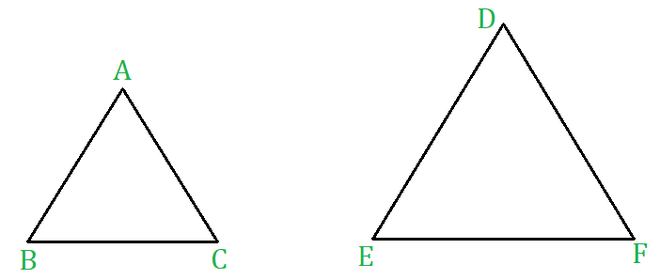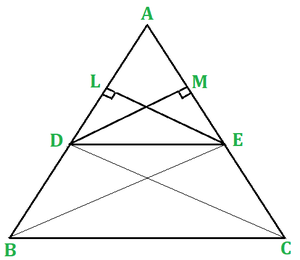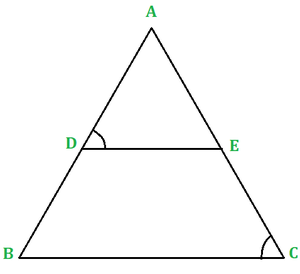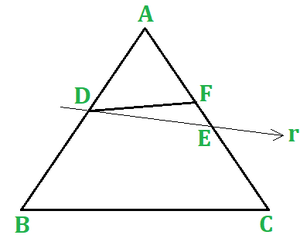GFG App
Open AppBrowser
Continue

Geometric figures which have the same shape but different sizes are known as similar figures. Two congruent figures are always similar but two similar figures need not be congruent.

Examples

(i) Any two line segments are similar.

(ii) Any two equivalent triangles are similar.

(iii) Any two rectangles are similar.

(iv) Any two squares are similar.

(v) Any two circles are similar.

### Similar Triangles

Two triangles are said to be similar to each other if

• their corresponding angles are equal, and
• their corresponding sides are proportional.Similar Triangles

In △ABC and △DEF if  (i) ∠A = ∠D , ∠B = ∠E, ∠C = ∠F

and (ii) AB/DE = BC/EF = CA/FD

Then above two triangles are similar, i.e. △ABC ∼ △DEF.

## Basic Proportionality Theorem or Thales‘ Theorem

Theorem: If a line is drawn parallel to one side of a triangle to intersect the other two sides in distinct points then the other two sides are divided in the same ratio.Proof:

Given: A △ABC in which DE || BC and DE intersects AB and AC at D and E respectively.

Construction: Join BE and CD. Draw EL ⟂ AB and DM ⟂ AC.

area (△DBE) = 1/2(DB x EL)

Again, area(△ADE) = area(△AED) = (1/2x AE x DM)

area(△ECD) = (1/2x EC x DM)

∴ area(△ADE)/area(△ECD) = (1/2 x AE x DM)/(1/2 x EC x DM) = AE/EC       ……….(ii)

Now △DBE and △ECD being on the same base DE and between the same parallels DE and BC , we have:

area(△DBE)/area(△ECD)         ……….(iii)

From eqn(i) and eqn(ii)

### Examples Based on Thales’ TheoremFigure 1

Example 1: In the given figure 1, DE || BC. If AD = 2.5cm, DB = 3cm, and AE = 3.75cm, Find AC?

Solution:

In △ABC, DE || BC

AD/DB = AE/EC   (By Thales’ Theorem)

⇒ 2.5/3 = 3.75/x, where EC = x cm

⇒ (3 * 3.75)/2.5 = 9/2 = 4.5

⇒ EC = 4.5cm

Hence, AC = (AE + EC) = 3.75 + 4.5 = 8.25 cm.

Example 2: In the figure 1 DE || BC.AD = 1.7cm, AB = 6.8cm, and AC = 9cm, Find AE?

Solution:

Let AE = x cm.

In △ABC, DE || BC

By Thales Theorem we have,

⇒ 1.7/6.8 = x/9

⇒ x = (1.7*9)/6.8 = 2.25

⇒ AE = 2.25cm

Hence AE = 2.25cm

Example 3: Prove that a line drawn through the midpoint of one side of a triangle (figure 1) parallel to another side bisects the third side.

Solution:

Given a ΔΑΒC in which D is the midpoint of AB and DE || BC, meeting AC at E.

TO PROVE AE = EC.

Proof: Since DE || BC, by Thales’ theorem, we have:

AE/EC = 1

⇒ AE = EC.

## The Converse of Thales’ Theorem

Theorem: (Converse of Thales’ theorem) If a line divides any two sides of triangle in the same ratio then the line must be parallel to the third side.Proof:

Given: A △ABC and a line r intersecting AB at D and AC at E, such that AD/DB = AE/EC

To prove: DE || BC

Proof: If possible, let DE not be parallel to BC. Then, there must be another line through D, which is parallel to BC.

Let DF || BC

Then by Thales Theorem,

But, AD/DB = AE/EC (given) ……..(ii)

From eqn(i) and eqn(ii)

AF/FC = AE/EC ⇒  AF/FC + 1 = AE/EC + 1

(AF + FC)/FC = (AE + EC)/EC

AC/FC = AC/EC ⇒ 1/FC = 1/EC

⇒ FC = EC.

This is possible only when E and F coincide.

Hence, DE || BC.

### Examples Based on Converse of Thales’ TheoremFigure 2

Example 1: In the given figure 2, AD/DB = AE/EC and ∠ADE = ∠ACB. Prove that ABC is an isosceles triangle.

Solution:

We have AD/DB = AE/EC ⇒ DE || BC [by the converse of Thales’ theorem]

Hence, ∠ABC = ∠ACB.

So, AB = AC [sides opposite to equal angles].

Hence, △ABC is an isosceles triangle.

Example 2: If D and E are points on the sides AB and AC respectively of △ABC  (figure 2) such that AB = 5.6cm, AD = 1.4cm, AC = 7.2cm, and AE = 1.8cm, show that DE || BC.

Solution:

Given, AB = 5.6cm, AD = 1.4cm, AC = 7.2cm and AE = 1.8cm

AD/AB = 1.4/5.6 = 1/4 and AE/AC = 1.8/7.2 = 1/4

Hence, by converse of Thales Theorem, DE || BC.

Example 3: Prove that the line segment joining the midpoints of any two sides of a triangle (figure 2) is parallel to the third side.

Solution:

In △ABC in which D and E are the midpoints of AB and AC respectively.

Since D and E are the midpoints of AB and AC respectively, we have :

AD = DB and AE = EC.

AD/DB = AE/EC (each equal to 1)

Hence, by converse of Thales Theorem, DE || BC

My Personal Notes arrow_drop_up